# MCAT Physical : Power

## Example Questions

### Example Question #81 : Mcat Physical Sciences

Two children are playing on an icy lake. Child 1 weighs 50kg, and child 2 weighs 38kg. Child 1 has a backpack that weighs 10kg, and child 2 has a backpack that weighs 5kg.

Over the course of the afternoon, they collide many times. Four collisions are described below.

Collision 1:

Child 1 starts from the top of a ramp, and after going down, reaches the lake surface while going 5m/s and subsequently slides into a stationary child 2. They remain linked together after the collision.

Collision 2:

Child 1 and child 2 are sliding in the same direction. Child 2, moving at 10m/s, slides into child 1, moving at 2m/s.

Collision 3:

The two children collide while traveling in opposite directions at 10m/s each.

Collision 4:

The two children push off from one another’s back, and begin moving in exactly opposite directions. Child 2 moves with a velocity of +8m/s.

After child 1 comes to a full stop in the above collisions, she decides she wants to keep going. If she sits on a sled and moves across the lake surface by pushing off the lake surface with her feet, which scenario below will have her producing the most power? Assume the sled has a mass of 10kg.

She can't produce net power unless there is an external input of energy

She goes from 2m/s to 4m/s in 2s

She goes from 2m/s to 5m/s in 6s

She goes from 2m/s to 5m/s in 4s

She goes from 2m/s to 4m/s in 1s

She goes from 2m/s to 4m/s in 1s

Explanation:

Power is equal to work/time. The work that child 1 is performing is also equal to the change in kinetic energy. When she goes from 2m/s to 4m/s, she is increasing her kinetic energy by mv2.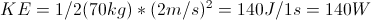This is the higest number of all the given scencarios.  To arrive at this conclusion, you must compare calculations for the other given scenarios.

### Example Question #12 : Work, Energy, And Power

A student holds a 10kg stack of books 1.5m above the ground for 20s. How much power was used to hold the books during this time?

0W

300W

7.35W

15W

147W

0W

Explanation:

Power is given by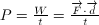. Although the student exerts a force on the books during this time, they are not moved at all, so the displacement d is 0, and thus the power is also 0.

### Example Question #11 : Work, Energy, And Power

A 100-Watt lightbulb is 3m away from a window with height 0.5m and width 0.5m. At what rate does the bulb's energy exit the window? Assume the light spreads out evenly in all directions.

2.65J/s

1.77J/s

0.88J/s

8.33J/s

3.54J/s

0.88J/s

Explanation:

First we can find the intensity of light in Watts per square meter, when the light reaches the window, by using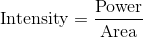.

Assuming the light spreads out evenly in all directions, it has spread out over the area of a sphere of radius 3m by the time it reaches the window.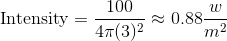Multiplying this intensity times the area of the window gives the power (or energy per second) leaving the window.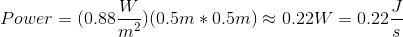### Example Question #4 : Power

Which of the following CANNOT be a unit for power?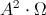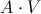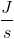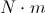Explanation:

In physics, power is often given the unit, W, or watts. In mechanics, a watt often represents the amount of work done over a unit of time, or Joules per second.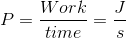In electrostatics a watt is expressed as the amount of current times the voltage, or as the current squared times the resistance.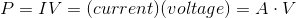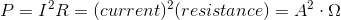A Newton-meter is a measure of energy or work, and can be written as a Joule, and is therefore the correct answer because it is not a measure of power.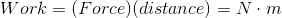### Example Question #5 : Power

An elevator lifts 100kg a distance of ten meters in a time of four seconds. What is the power of the elevator?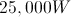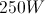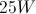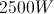Explanation:

The power of an elevator can be determined using the equation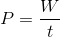, with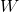being the work done by the elevator, andbeing the time in which energy is transferred. Using this, we can plug in the known values and solve for  the power.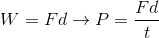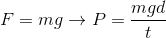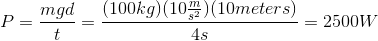### Example Question #6 : Power

Two students (student X and student Y) lift a boulder vertically from point A to point B. Student X directly lifts the boulder from point A to point B, whereas student Y uses a pulley to lift the boulder. This allows student Y to apply a force (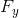) that is three times smaller than the force applied by student X (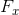). Both students apply force upwards and take the same amount of time to complete this task.

The vertical distance between point A and point B is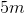.

The power exerted by student Y is __________.

equal to the force exerted by student Y times five

equal to force exerted by student Y times two

less than the power exerted by student X

equal to the power exerted by student X

less than the power exerted by student X

Explanation:

To answer this question you need to know the definitions of work and power: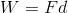The units of work and power are Joules and watts, respectively. The passage states that the amount of time it takes to complete the task is same for both students; therefore, you can ignore the effects of time on power.

The passage also states that student Y applies less force. Since student Y applies less force, he has to expend less energy (work) than student X. This means that student Y also has to exert less power. A reduction in force will reduce work, and subsequently reduce power. We can thus conclude that student Y exerts less power than student X.

### Example Question #7 : Power

Two students (student X and student Y) lift a boulder vertically from point A to point B. Student X directly lifts the boulder from point A to point B, whereas student Y uses a pulley to lift the boulder. This allows student Y to apply a force () that is three times smaller than the force applied by student X (). Both students apply force upwards and take the same amount of time to complete this task.

The vertical distance between point A and point B is.

If student Y exerts a force of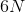and completes the task in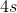. What is the power exerted by student X?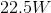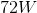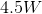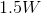Explanation:

The first step is to find the force exerted by student X. The passage states that student Y exerts three times less force than student X; therefore, student X exerts three times more than student Y: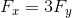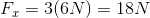The second step is to find the amount of work done by student X.Remember that student X directly lifts the boulder from point A to point B. This means that the distance the boulder travels is the vertical distance between point A and B ().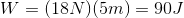The question states that time for the entire process is. Since the time is the same for both students, power exerted by student X is: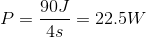The correct answer is.